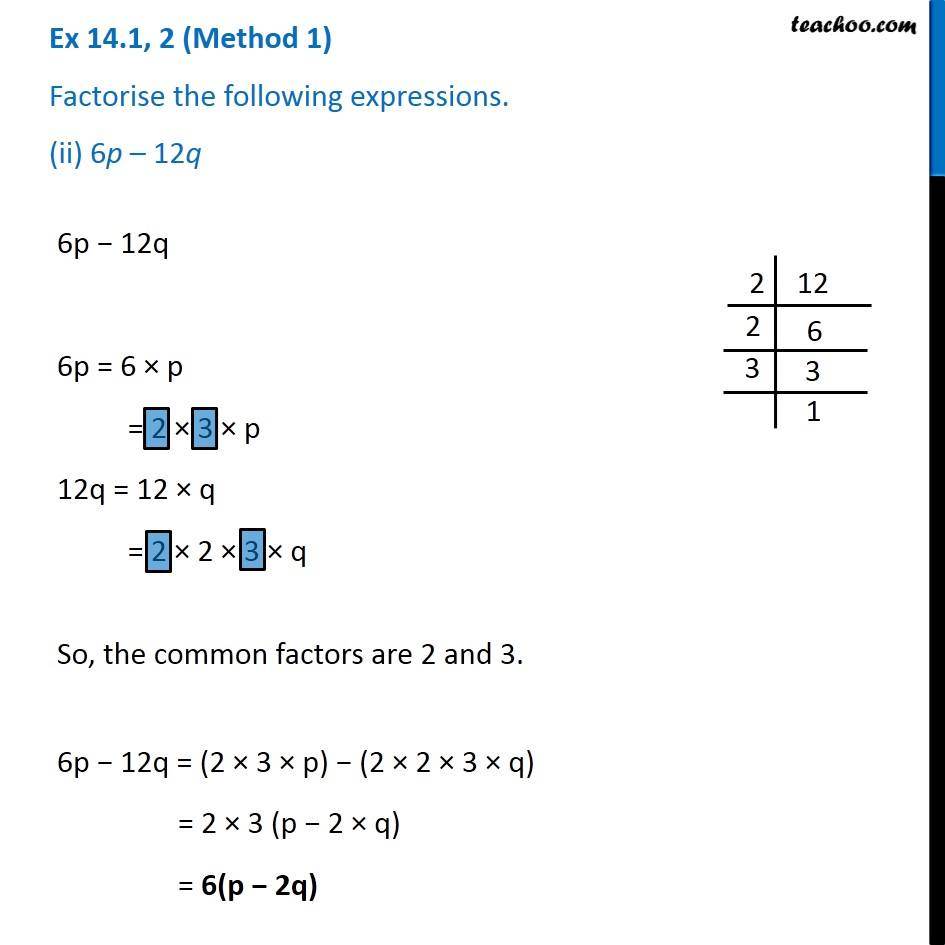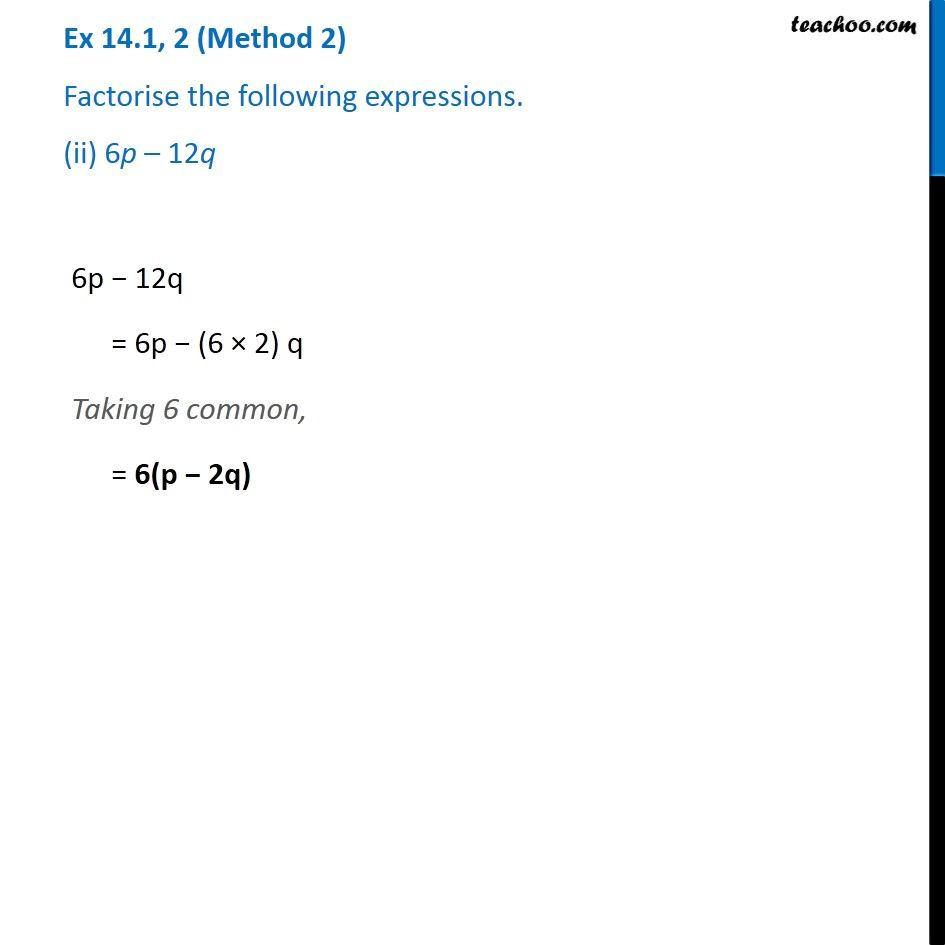Factorisation using common factors

Chapter 12 Class 8 Factorisation
Concept wiseLearn in your speed, with individual attention - Teachoo Maths 1-on-1 Class

### Transcript

Ex 12.1, 2 (Method 1) Factorise the following expressions. (ii) 6p – 12q 6p − 12q 6p = 6 × p = 2 × 3 × p 12q = 12 × q = 2 × 2 × 3 × q So, the common factors are 2 and 3. 6p − 12q = (2 × 3 × p) − (2 × 2 × 3 × q) = 2 × 3 (p − 2 × q) = 6(p − 2q) Ex 12.1, 2 (Method 2) Factorise the following expressions. (ii) 6p – 12q 6p − 12q = 6p − (6 × 2) q Taking 6 common, = 6(p − 2q)